# Ensemble sorting of a 3Brain Biocam recording from a retina

## Ensemble sorting of a 3Brain Biocam recording from a mouse retina¶

This notebook reproduces supplemental figure S3 from the paper SpikeInterface, a unified framework for spike sorting.

The recording was made by Gerrit Hilgen in the lab of Evelyne Sernagor, University of Newcastle.

The data set for this notebook is available on the Dandi Archive: https://gui.dandiarchive.org/#/dandiset/000034

The entire data archive can be downloaded with the command `dandi download https://gui.dandiarchive.org/#/dandiset/000034/draft` (about 75GB).

File required to run the code:

The file should be in the same directory where the notebook is located (otherwise adjust paths below).

Author: Matthias Hennig, University of Edinburgh, 24 Aug 2020

#### Requirements¶

For this need you will need the following Python packages:

• numpy
• pandas
• matplotlib
• seaborn
• spikeinterface
• dandi

To run the MATLAB-based sorters, you would also need a MATLAB license. For other sorters, please refer to the documentation on how to install sorters.

In :
```import os

# Matlab sorter paths:
# change these to match your environment
os.environ["IRONCLUST_PATH"] = "./ironclust"
os.environ["KILOSORT2_PATH"] = "./Kilosort2"
os.environ["HDSORT_PATH"] = "./HDsort"

import matplotlib.pyplot as plt
import numpy as np
from pathlib import Path
import pandas as pd
import seaborn as sns
from collections import defaultdict
from matplotlib_venn import venn3

import spikeinterface as si
import spikeextractors as se
import spiketoolkit as st
import spikesorters as ss
import spikecomparison as sc
import spikewidgets as sw
from spikecomparison import GroundTruthStudy, MultiSortingComparison

%matplotlib inline

def clear_axes(ax):
ax.spines['top'].set_visible(False)
ax.spines['right'].set_visible(False)

# print version information
si.print_spikeinterface_version()
ss.print_sorter_versions()
```
```spikeinterface: 0.9.9
* spikeextractor: 0.8.4
* spiketoolkit: 0.6.3
* spikesorters: 0.3.3
* spikecomparison: 0.2.6
* spikewidgets: 0.4.3

hdsort: version = '1.0.2'

herdingspikes: 0.3.7
ironclust: 5.9.8
klusta: 3.0.16
mountainsort4: unknown
spykingcircus: 0.9.7
tridesclous: 1.6.0
```
In :
```# the recording file, downloaded from Dandi in NWB:N format
data_file =  './sub-P29-16-05-14-retina02-left_ecephys.nwb'

# paths for spike sorter outputs
p = Path('./')

# select spike sorters to be used, note Kilosort2 requires a NVIDIA GPU to run
sorter_list = ['herdingspikes', 'kilosort2', 'ironclust', 'hdsort']
sorter_names = ['HerdingSpikes', 'Kilosort2', 'Ironclust',  'HDSort']
sorter_names_short = ['HS', 'KS', 'IC', 'HDS']

# create a recording extractor, this gives access to the raw data in the NWB:N file
recording = se.NwbRecordingExtractor(data_file)

# this recording has some bad artefcats, and these are easily blanked out using spiketoolkit
# note that no spike sorter can handle these artefacts well, so this step is essential
recording = st.preprocessing.blank_saturation(recording)
# (aside: the warning below can be ignored, artefacts are quite sparse but definetely there, the check
# if very conservative)

# NWB:N files store the data in (channels:time) order, but for spike sorting the transposed format is much
# more efficient. Therefore here we create a CacheRecordingExtractor that re-writes the data
# as a binary file in the desired order. This will take some time, but speeds up subsequent steps:
recording = se.CacheRecordingExtractor(recording)

# print some info
print("Sampling rate: {}Hz".format(recording.get_sampling_frequency()))
print("Duration: {}s".format(recording.get_num_frames()/recording.get_sampling_frequency()))
print("Number of channels: {}".format(recording.get_num_channels()))
```
```Warning, narrow signal range suggests artefact-free data.
Sampling rate: 23199.090358491783Hz
Duration: 135.7303218075276s
Number of channels: 1024
```

## Run spike sorters and perform comparison between all outputs¶

In :
```# now create the study environment and run all spike sorters
# note that this function will not re-run a spike sorter if the sorting is already present in
# the working folder

study_folder = p / 'study/'
working_folder = p / 'working/'
if not study_folder.is_dir():
print('Setting up study folder:', study_folder)
os.mkdir(study_folder)
rec_dict = {'rec': recording}

result_dict = ss.run_sorters(sorter_list=sorter_list, recording_dict_or_list=rec_dict, with_output=True,
sorter_params=sorter_params, working_folder=working_folder, engine='loop',
mode='keep', verbose=True)

# when done, load all sortings into a handly list
sortings = []
for s in sorter_list:
sortings.append(result_dict['rec',s])
```
```/disk/scratch/mhennig/miniconda3/envs/spikesorting/lib/python3.7/site-packages/spikeextractors/extractors/matsortingextractor/matsortingextractor.py:65: ResourceWarning: unclosed file <_io.BufferedReader name='/disk/scratch/mhennig/spikeinterface/retina_short/working/rec/hdsort/hdsort_output/hdsort_output_results.mat'>
raise ImportError("Version 7.2 .mat file given, but you don't have h5py installed.")
ResourceWarning: Enable tracemalloc to get the object allocation traceback
```
In :
```# run a multi-sorting comparison, an all-to-all comparison
# results are saved and just loaded from a file if this exists

if not os.path.isfile(study_folder / 'multicomparison.gpickle'):
mcmp = sc.compare_multiple_sorters(sorting_list=sortings, name_list=sorter_names_short,
verbose=True)
print('saving multicomparison')
mcmp.dump(study_folder)
else:
```
```loading multicomparison
Multicomaprison step 3: clean graph
Removed edge ('KS_244', 'HS_18', {'weight': 0.6169491525423729})
Removed edge ('IC_181', 'HDS_137003', {'weight': 0.9284436493738819})
Removed edge ('HDS_450004', 'KS_1160', {'weight': 0.5208791208791209})
Removed 3 duplicate nodes
Multicomaprison step 4: extract agreement from graph
```
In :
```# plot an activity map
# as the channel count is high, here we use the spikes detected by HS2

sx = result_dict['rec','herdingspikes']
n,v = np.histogram(sx._rf['ch'], bins=np.arange(1025))

ax = plt.subplot(111)
ax.imshow(n.reshape((32,32)))
ax.set_xticks(())
ax.set_yticks(())
ax.plot((10,10+100/42),(-1,-1),'k-')
ax.annotate('100\$\\mu m\$',(10+100/42/2,-2), ha='center');
ax.axis('off')
ax.set_aspect('equal')
```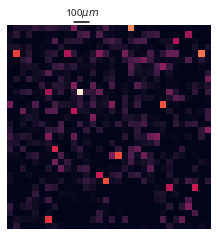In :
```# next we plot some raw data traces
# for better visualisation, we bandpass filter the traces before showing them
# to this end, we use a lazy bandpass filter from spiketoolkit
recording_bandpass = st.preprocessing.bandpass_filter(recording)
plt.figure(figsize=(12,3))
ax = plt.subplot(111)
w = sw.plot_timeseries(recording_bandpass, channel_ids=range(300,308), color='k', ax=ax, trange=(2,3))
ax.plot((2.01,2.11),(-50,-50),'k-');
ax.annotate('100ms',(2.051,-120), ha='center');
ax.axis('off');
```
```Warning: dumping a CacheRecordingExtractor. The path to the tmp binary file will be lost in further sessions. To prevent this, use the 'CacheRecordingExtractor.move_to('path-to-file)' function
```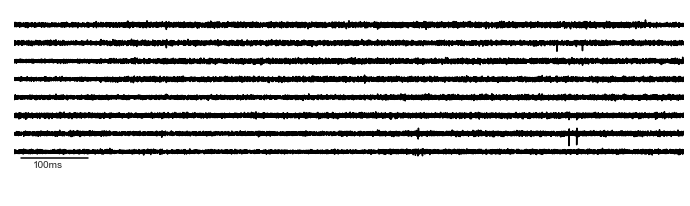In :
```# number of units found by each sorter
ax = plt.subplot(111)
ax.bar(range(len(sortings)), [len(s.get_unit_ids()) for s in sortings])
ax.set_xticks(range(len(sorter_names)))
ax.set_xticklabels(sorter_names_short, rotation=60, ha='center')
ax.set_ylabel('Units detected')
```
Out:
`Text(0, 0.5, 'Units detected')`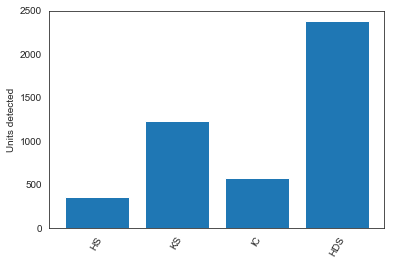In :
```# spikewidgets provides handy widgets to plot summary statistics of the comparison

# show the number of units agreed upon by k sorters, in aggregate
plt.figure()
ax = plt.subplot(111)
w = sw.plot_multicomp_agreement(mcmp, plot_type='pie', ax=ax)

# show the number of units agreed upon by k sorters, per sorter
plt.figure()
ax = plt.subplot(111)
w = sw.plot_multicomp_agreement_by_sorter(mcmp, show_legend=True, ax=ax)
```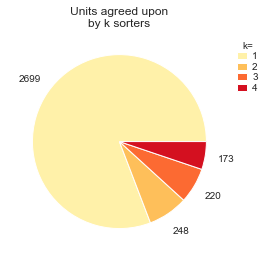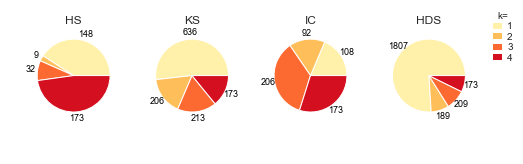# Ensemble sorting of a Neuropixels recording 2

## Ensemble sorting of a Neuropixel recording (2)¶

This notebook reproduces supplemental figure S2 from the paper SpikeInterface, a unified framework for spike sorting.

The recording was made by André Marques-Smith in the lab of Adam Kampff. Reference:

Marques-Smith, A., Neto, J.P., Lopes, G., Nogueira, J., Calcaterra, L., Frazão, J., Kim, D., Phillips, M., Dimitriadis, G., Kampff, A.R. (2018). Recording from the same neuron with high-density CMOS probes and patch-clamp: a ground-truth dataset and an experiment in collaboration. bioRxiv 370080; doi: https://doi.org/10.1101/370080

The data set for this notebook is available on the Dandi Archive: https://gui.dandiarchive.org/#/dandiset/000034

The entire data archive can be downloaded with the command `dandi download https://gui.dandiarchive.org/#/dandiset/000034/draft` (about 75GB).

File required to run the code:

This file should be in the same directory where the notebook is located (otherwise adjust paths below).

Author: Matthias Hennig, University of Edinburgh, 25 Aug 2020

#### Requirements¶

For this need you will need the following Python packages:

• numpy
• pandas
• matplotlib
• seaborn
• spikeinterface
• dandi

To run the MATLAB-based sorters, you would also need a MATLAB license. For other sorters, please refer to the documentation on how to install sorters.

In :
```import os

# Matlab sorter paths:
# change these to match your environment
os.environ["IRONCLUST_PATH"] = "./ironclust"
os.environ["KILOSORT2_PATH"] = "./Kilosort2"
os.environ["HDSORT_PATH"] = "./HDsort"

import matplotlib.pyplot as plt
import numpy as np
from pathlib import Path
import pandas as pd
import seaborn as sns
from collections import defaultdict
from matplotlib_venn import venn3

import spikeinterface as si
import spikeextractors as se
import spiketoolkit as st
import spikesorters as ss
import spikecomparison as sc
import spikewidgets as sw
from spikecomparison import GroundTruthStudy, MultiSortingComparison

%matplotlib inline

def clear_axes(ax):
ax.spines['top'].set_visible(False)
ax.spines['right'].set_visible(False)

# print version information
si.print_spikeinterface_version()
ss.print_sorter_versions()
```
```spikeinterface: 0.9.9
* spikeextractor: 0.8.4
* spiketoolkit: 0.6.3
* spikesorters: 0.3.3
* spikecomparison: 0.2.6
* spikewidgets: 0.4.3

hdsort: version = '1.0.2'

herdingspikes: 0.3.7
ironclust: 5.9.8
klusta: 3.0.16
mountainsort4: unknown
spykingcircus: 0.9.7
tridesclous: 1.6.0
```
In :
```# the recording file, downloaded from Dandi in NWB:N format
data_file =  './sub-c1_ecephys.nwb'

# paths for spike sorter outputs
p = Path('./')

# select spike sorters to be used, note Kilosort2 requires a NVIDIA GPU to run
sorter_list = ['herdingspikes', 'kilosort2', 'ironclust', 'tridesclous', 'spykingcircus', 'hdsort']
sorter_params = {
#     'kilosort2': {'keep_good_only': True}, # removes good units!
'herdingspikes': {'filter': True,
}
}
sorter_names = ['HerdingSpikes', 'Kilosort2', 'Ironclust','Tridesclous', 'SpykingCircus', 'HDSort']
sorter_names_short = ['HS', 'KS', 'IC', 'TDC', 'SC', 'HDS']

# create a recording extractor, this gives access to the raw data in the NWB:N file
recording = se.NwbRecordingExtractor(data_file)

# NWB:N files store the data in (channels:time) order, but for spike sorting the transposed format is much
# more efficient. Therefore here we can create a CacheRecordingExtractor that re-writes the data
# as a binary file in the desired order. This will take some time, but speeds up subsequent steps:
# recording = se.CacheRecordingExtractor(recording)

# print some info
print("Sampling rate: {}Hz".format(recording.get_sampling_frequency()))
print("Duration: {}s".format(recording.get_num_frames()/recording.get_sampling_frequency()))
print("Number of channels: {}".format(recording.get_num_channels()))
```
```Sampling rate: 30000.0Hz
Duration: 270.01123333333334s
Number of channels: 384
```

## Run spike sorters and perform comparison between all outputs¶

In :
```# now create the study environment and run all spike sorters
# note that this function will not re-run a spike sorter if the sorting is already present in
# the working folder

study_folder = p / 'study/'
working_folder = p / 'working/'
if not study_folder.is_dir():
print('Setting up study folder:', study_folder)
os.mkdir(study_folder)
rec_dict = {'rec': recording}

result_dict = ss.run_sorters(sorter_list=sorter_list, recording_dict_or_list=rec_dict, with_output=True,
sorter_params=sorter_params, working_folder=working_folder, engine='loop',
mode='keep', verbose=True)

# when done, load all sortings into a handly list
sortings = []
for s in sorter_list:
sortings.append(result_dict['rec',s])
```
```/disk/scratch/mhennig/miniconda3/envs/spikesorting/lib/python3.7/site-packages/spikeextractors/extractors/matsortingextractor/matsortingextractor.py:65: ResourceWarning: unclosed file <_io.BufferedReader name='/disk/scratch/mhennig/spikeinterface/neuropixels_ms/working/rec/hdsort/hdsort_output/hdsort_output_results.mat'>
raise ImportError("Version 7.2 .mat file given, but you don't have h5py installed.")
ResourceWarning: Enable tracemalloc to get the object allocation traceback
```
In :
```# run a multi-sorting comparison, an all-to-all comparison
# results are saved and just loaded from a file if this exists

if not os.path.isfile(study_folder / 'multicomparison.gpickle'):
mcmp = sc.compare_multiple_sorters(sorting_list=sortings, name_list=sorter_names_short,
verbose=True)
print('saving multicomparison')
mcmp.dump(study_folder)
else:
```
```loading multicomparison
Multicomaprison step 3: clean graph
Removed edge ('HDS_25017', 'KS_231', {'weight': 0.5272206303724928})
Removed edge ('IC_66', 'TDC_28', {'weight': 0.5032594524119948})
Removed edge ('KS_231', 'HS_9', {'weight': 0.5059360730593607})
Removed edge ('SC_119', 'TDC_28', {'weight': 0.5013054830287206})
Removed edge ('KS_71', 'HS_57', {'weight': 0.5238095238095238})
Removed 5 duplicate nodes
Multicomaprison step 4: extract agreement from graph
```
In :
```# plot an activity map
# the method uses a rather simpe (and slow) threshold spike detection

plt.figure(figsize=(16,2))
ax = plt.subplot(111)
w = sw.plot_activity_map(recording, transpose=True, ax=ax, background='w', frame=True)
ax.plot((50,150),(-40,-40),'k-')
ax.annotate('100\$\\mu m\$',(100,-115), ha='center');
```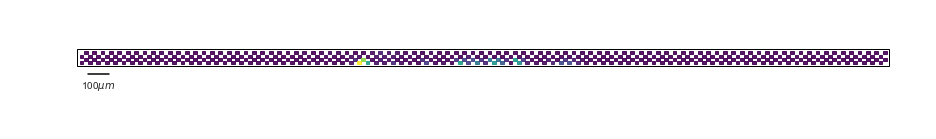In :
```# raw data traces

plt.figure(figsize=(12,3))
ax = plt.subplot(111)
w = sw.plot_timeseries(recording, channel_ids=range(160,168), color='k', ax=ax, trange=(7,8))
ax.axis('off')
ax.plot((7.01,7.11),(20,20),'k-')
ax.annotate('100ms',(7.051,-190), ha='center');
```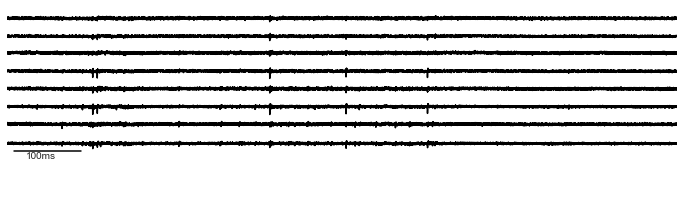In :
```# number of units found by each sorter

ax = plt.subplot(111)
ax.bar(range(len(sortings)), [len(s.get_unit_ids()) for s in sortings])
ax.set_xticks(range(len(sorter_names)))
ax.set_xticklabels(sorter_names_short, rotation=60, ha='center')
ax.set_ylabel('Units detected')
```
Out:
`Text(0, 0.5, 'Units detected')`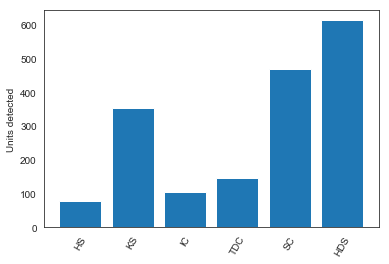In :
```# spikewidgets provides handy widgets to plot summary statistics of the comparison

# show the number of units agreed upon by k sorters, in aggregate
plt.figure()
ax = plt.subplot(111)
w = sw.plot_multicomp_agreement(mcmp, plot_type='pie', ax=ax)

# show the number of units agreed upon by k sorters, per sorter
plt.figure()
ax = plt.subplot(111)
w = sw.plot_multicomp_agreement_by_sorter(mcmp, show_legend=True, ax=ax)
```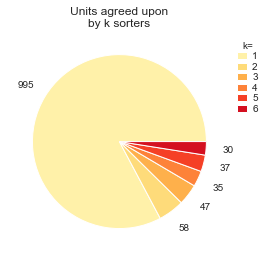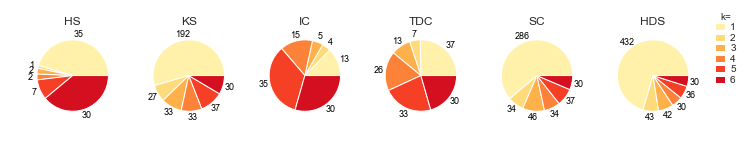# Ground truth comparison and ensemble sorting of a synthetic Neuropixels recording

## Ground-truth comparison and ensemble sorting of a synthetic Neuropixels recording¶

This notebook reproduces figures 2 and 3 from the paper SpikeInterface, a unified framework for spike sorting.

The data set for this notebook is available on the Dandi Archive: https://gui.dandiarchive.org/#/dandiset/000034.

The entire data archive can be downloaded with the command `dandi download https://gui.dandiarchive.org/#/dandiset/000034/draft` (about 75GB).

The data file required to run the code is:

This file should be in the same directory where the notebook is located (otherwise adjust paths below).

Author: Matthias Hennig, University of Edinburgh, 22 Aug 2020

#### Requirements¶

For this need you will need the following Python packages:

• numpy
• pandas
• matplotlib
• seaborn
• spikeinterface
• dandi
• matplotlib-venn

To run the MATLAB-based sorters, you would also need a MATLAB license. For other sorters, please refer to the documentation on how to install sorters.

In :
```import os

# Matlab sorter paths:
# change these to match your environment
os.environ["IRONCLUST_PATH"] = "./ironclust"
os.environ["KILOSORT2_PATH"] = "./Kilosort2"
os.environ["HDSORT_PATH"] = "./HDsort"

import matplotlib.pyplot as plt
import numpy as np
from pathlib import Path
import pandas as pd
import seaborn as sns
from collections import defaultdict
from matplotlib_venn import venn3

import spikeinterface as si
import spikeextractors as se
import spiketoolkit as st
import spikesorters as ss
import spikecomparison as sc
import spikewidgets as sw
from spikecomparison import GroundTruthStudy

%matplotlib inline

def clear_axes(ax):
ax.spines['top'].set_visible(False)
ax.spines['right'].set_visible(False)

# print version information
si.print_spikeinterface_version()
ss.print_sorter_versions()
```
```spikeinterface: 0.9.9
* spikeextractor: 0.8.4
* spiketoolkit: 0.6.3
* spikesorters: 0.3.3
* spikecomparison: 0.2.6
* spikewidgets: 0.4.3

hdsort: version = '1.0.2'

herdingspikes: 0.3.7
ironclust: 5.9.8
klusta: 3.0.16
mountainsort4: unknown
spykingcircus: 0.9.7
tridesclous: 1.6.0
```

## Set up ground truth study an run all sorters¶

In :
```study_path = Path('.')
data_path = Path('.')
study_folder = study_path / 'study_mearec_250cells_Neuropixels-384chans_duration600s_noise10uV_2020-02-28/'

# the original data
# this NWB file contains both the ground truth spikes and the raw data
data_filename = data_path / 'sub-MEAREC-250neuron-Neuropixels_ecephys.nwb'
SX_gt = se.NwbSortingExtractor(str(data_filename))
RX = se.NwbRecordingExtractor(str(data_filename))

if not os.path.isdir(study_folder):
gt_dict = {'rec0' : (RX, SX_gt) }
study = GroundTruthStudy.create(study_folder, gt_dict)
else:
study = GroundTruthStudy(study_folder)

sorter_list = ['herdingspikes', 'kilosort2', 'ironclust',
'spykingcircus', 'tridesclous', 'hdsort']
sorter_names = ['HerdingSpikes', 'Kilosort2', 'Ironclust',
'SpykingCircus', 'Tridesclous', 'HDSort']
sorter_names_short = ['HS', 'KS', 'IC', 'SC', 'TDC', 'HDS']

study.run_sorters(sorter_list, mode='keep', engine='loop', verbose=True)

study.copy_sortings()

# compute or load SNR for the ground truth units
snr_file = study_folder / 'snr.npy'
if os.path.isfile(snr_file):
else:
print('computing snr')
# note this is quite slow for a NWB file as the data is arranged as channels:time
# it is faster to first write out a binary file in time:channels order
snr = st.validation.compute_snrs(SX_gt, RX, apply_filter=False, verbose=False,
memmap=True, max_spikes_per_unit_for_snr=500)
np.save(snr_file, snr)
```
```/disk/scratch/mhennig/miniconda3/envs/spikesorting/lib/python3.7/site-packages/spikeextractors/extractors/matsortingextractor/matsortingextractor.py:65: ResourceWarning: unclosed file <_io.BufferedReader name='/disk/scratch/mhennig/spikeinterface/paper/MEArec/study_mearec_250cells_Neuropixels-384chans_duration600s_noise10uV_2020-02-28/sorter_folders/rec0/hdsort/hdsort_output/hdsort_output_results.mat'>
raise ImportError("Version 7.2 .mat file given, but you don't have h5py installed.")
ResourceWarning: Enable tracemalloc to get the object allocation traceback
```

## Run the ground truth comparison and summarise the results¶

In :
```study.run_comparisons(exhaustive_gt=True, match_score=0.1)
comparisons = study.comparisons
dataframes = study.aggregate_dataframes()
```
In :
```# comparison summary
dataframes['count_units']
```
Out:
rec_name sorter_name num_gt num_sorter num_well_detected num_redundant num_overmerged num_false_positive num_bad
0 rec0 ironclust 250 283 202 8 2 41 49
1 rec0 spykingcircus 250 343 175 43 1 56 99
2 rec0 tridesclous 250 189 135 3 5 9 12
3 rec0 hdsort 250 457 196 4 3 210 214
4 rec0 kilosort2 250 415 245 21 2 147 168
5 rec0 herdingspikes 250 233 128 6 3 39 45

## Figure 1 - ground truth study results¶

In :
```# activity levels on the probe

plt.figure(figsize=(16,2))
ax = plt.subplot(111)
w = sw.plot_activity_map(RX, trange=(0,20), transpose=True, ax=ax, background='w', frame=True)
ax.plot((-1800,-1700), (-120,-120), 'k-')
ax.annotate('100\$\\mu m\$',(-1750,-220), ha='center');
```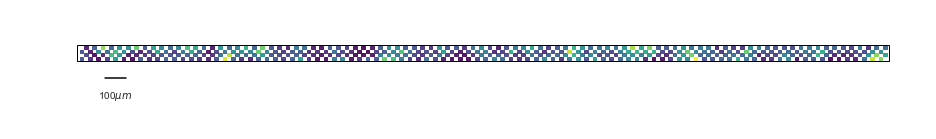In :
```# example data traces

plt.figure(figsize=(16,6))
ax = plt.subplot(111)
w = sw.plot_timeseries(RX, channel_ids=range(10,18), color='k', ax=ax, trange=(1,2))
ax.axis('off')
p = ax.get_position()
p.y0 = 0.58
ax.set_position(p)
ax.set_xticks(())
ax.plot((1.01,1.11),(-400,-400),'k-')
ax.annotate('100ms',(1.051,-750), ha='center');
ax.set_ylim((-750,ax.set_ylim()))
```
Out:
`(-750, 4435.259765625)`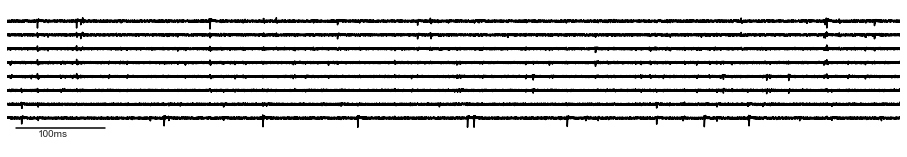In :
```ax = plt.subplot(111)
n = []
for s in sorter_list:
n.append(len(study.get_sorting(s).get_unit_ids()))
ax.bar(range(len(sorter_list)), n, color='tab:blue')
ax.set_xticks(range(len(sorter_names_short)))
ax.set_xticklabels(sorter_names_short, rotation=60, ha='center')
ax.set_ylabel('Units detected')
clear_axes(ax)
```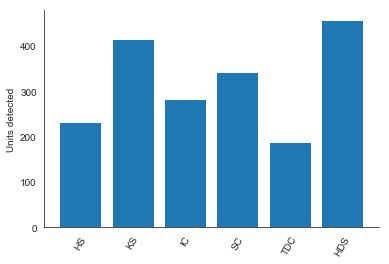In :
```ax = plt.subplot(111)
p = ax.get_position()
p.x1=0.85
ax.set_position(p)
sns.set_palette(sns.color_palette("Set1"))
df = pd.melt(dataframes['perf_by_units'], id_vars='sorter_name', var_name='Metric', value_name='Score',
value_vars=('accuracy','precision', 'recall'))
sns.swarmplot(data=df, x='sorter_name', y='Score', hue='Metric', dodge=True,
order=sorter_list,  s=3, ax=ax)
ax.set_xticklabels(sorter_names_short, rotation=30, ha='center')
ax.legend(bbox_to_anchor=(1.0, 1), loc=2, borderaxespad=0., frameon=False, fontsize=8, markerscale=0.5)
ax.set_xlabel(None);
ax.set_ylabel('Score');
clear_axes(ax)
```
```/disk/scratch/mhennig/miniconda3/envs/spikesorting/lib/python3.7/site-packages/matplotlib/axes/_axes.py:4204: DeprecationWarning: Using or importing the ABCs from 'collections' instead of from 'collections.abc' is deprecated, and in 3.8 it will stop working
(isinstance(c, collections.Iterable) and
```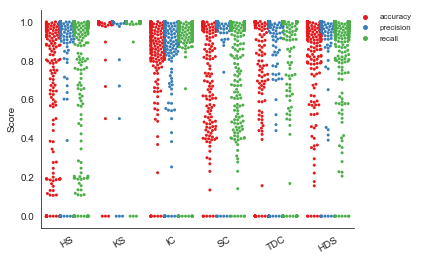In :
```ax = plt.subplot(111)
p = ax.get_position()
p.x1=0.85
ax.set_position(p)
df = pd.melt(dataframes['count_units'], id_vars='sorter_name', var_name='Type', value_name='Units',
value_vars=('num_well_detected', 'num_false_positive', 'num_redundant', 'num_overmerged'))
sns.set_palette(sns.color_palette("Set1"))
sns.barplot(x='sorter_name', y='Units', hue='Type', data=df,
order=sorter_list, ax=ax)
ax.set_xticklabels(sorter_names_short, rotation=30, ha='right')
ax.legend(bbox_to_anchor=(1.0, 1), loc=2, borderaxespad=0., frameon=False, fontsize=8, markerscale=0.1)
for t, l in zip(ax.legend_.texts, ("Well detected", "False positive", "Redundant", "Overmerged")): t.set_text(l)
ax.set_xlabel(None);
clear_axes(ax)
```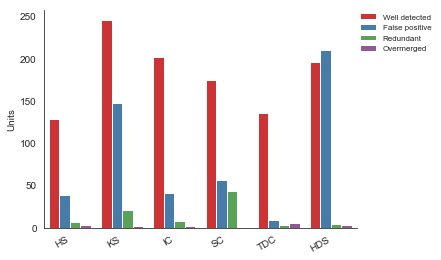In :
```# precision vs. recall and accuracy vs. SNR
fig = plt.figure(figsize=(14, 4))

sns.set_palette(sns.color_palette("deep"))

axesA = []
for i,s in enumerate(sorter_list):
ax = plt.subplot(2,len(sorter_list),i+1)
axesA.append(ax)

g = sns.scatterplot(data=dataframes['perf_by_units'].loc[dataframes['perf_by_units'].sorter_name==s],
x='precision', y='recall', s=30, edgecolor=None, alpha=0.1)
ax.set_title(sorter_names[i])
ax.set_aspect('equal')
clear_axes(ax)
ax.set_xlabel('Precision')
ax.set_ylabel('Recall')

for ax in axesA[1:]:
axesA.get_shared_y_axes().join(axesA, ax)
ax.set_yticklabels([])
ax.set_ylabel('')
ax.set_xlabel('')
ax.autoscale()

############### B

df = dataframes['perf_by_units']

# add snr to the by-unit table
if 'snr' not in df.keys():
snr_d = {k:snr[k] for i,k in enumerate(SX_gt.get_unit_ids())}
df['snr'] = df['gt_unit_id'].map(snr_d)

axesB = []
for i,s in enumerate(sorter_list):
ax = plt.subplot(2,len(sorter_list),len(sorter_list)+i+1)
axesB.append(ax)

g = sns.scatterplot(data=dataframes['perf_by_units'].loc[dataframes['perf_by_units'].sorter_name==s],
x='snr', y='accuracy', s=30, alpha=0.2)
clear_axes(ax)
ax.set_xlabel('Ground truth SNR')
ax.set_ylabel('Accuracy')

for ax in axesB[1:]:
axesB.get_shared_y_axes().join(axesB, ax)
ax.set_yticklabels([])
ax.set_ylabel('')
ax.set_xlabel('')
ax.autoscale()

```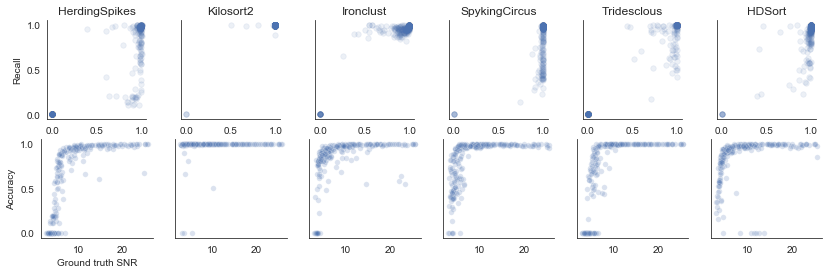## Figure 3 - comparison of sorter outputs and ensembe sorting¶

In :
```# perform an all-to-all multicomparison or load it from
# disk if file exists

sortings = []
for s in sorter_list:
sortings.append(study.get_sorting(s))

cmp_folder = study_folder / 'multicomparison/'
if not os.path.isdir(cmp_folder):
os.mkdir(cmp_folder)
if not os.path.isfile(cmp_folder / 'multicomparison.gpickle'):
mcmp = sc.compare_multiple_sorters(sorting_list=sortings, name_list=sorter_names_short,
verbose=False, match_score=0.5)
print('saving multicomparison')
mcmp.dump(cmp_folder)
else:

mcmp_graph = mcmp.graph.copy()
```
```loading multicomparison
```
In :
```# get sorting extractors with unit with no agreement (minimum agreement = 1) and one
# with at least 2 sorters in agreement
not_in_agreement = mcmp.get_agreement_sorting(minimum_agreement_count=1, minimum_agreement_count_only=True)
in_agreement = mcmp.get_agreement_sorting(minimum_agreement_count=2)

# score these against ground truth
cmp_no_agr = sc.compare_sorter_to_ground_truth(SX_gt, not_in_agreement)
cmp_agr = sc.compare_sorter_to_ground_truth(SX_gt, in_agreement)

# now collect results for each sorter:

# create dict to collect results
results = {'TP':{}, 'FP':{}, 'SNR':{}}
ns = len(sorter_names_short)
for s in sorter_names_short:
results['TP'][s] = dict(zip(range(1,ns+1), *(ns+1)))
results['FP'][s] = dict(zip(range(1,ns+1), *(ns+1)))
results['SNR'][s] = dict(zip(range(1,ns+1), [[]]*(ns+1)))

# sorter names
dict_names = dict(zip(sorter_names_short, sorter_list))

# iterate over all units gathered from subgraphs
for u in  mcmp._new_units.keys():
found_in_gt = []
gt_index = []
# check if units have a match in ground truth, store boolen
for u2 in mcmp._new_units[u]['sorter_unit_ids'].items():
found_in_gt.append(u2 in study.comparisons['rec0',dict_names[u2]].best_match_12.values)
if found_in_gt[-1]:
gt_index.append(np.where(study.comparisons['rec0',dict_names[u2]].best_match_12==u2))
if len(set(gt_index))>1:
print('different gt units: ',u, gt_index)
if np.sum(found_in_gt)==len(found_in_gt):
#     if np.sum(found_in_gt)>0:#==len(found_in_gt):  # use this if interested in equal matches
key = 'TP'
else:
key = 'FP'
if len(found_in_gt)>1:
print('FP unit found by >1 sorter: ',u)

for i,u2 in enumerate(mcmp._new_units[u]['sorter_unit_ids'].items()):
#         results[key][u2][np.sum(found_in_gt)] += 1 # use this if interested in equal matches
results[key][u2][len(found_in_gt)] += 1
if key is 'TP':
# something odd with nested oython dicts requires this:
d = results['SNR'][u2][len(found_in_gt)].copy()
d.append(snr[gt_index[i]])
results['SNR'][u2][len(found_in_gt)] = d
# this fails, I wonder why:
# results['SNR'][u2][len(found_in_gt)].append(snr[gt_index[i]])
```
```different gt units:  20 [213, 213, 213, 146, 213, 213]
different gt units:  35 [189, 189, 185, 189, 189]
different gt units:  42 [224, 224, 224, 76, 224, 224]
different gt units:  46 [108, 108, 108, 90, 108, 108]
FP unit found by >1 sorter:  92
different gt units:  102 [153, 197, 197, 197, 197, 197]
different gt units:  103 [175, 175, 175, 11, 175, 175]
FP unit found by >1 sorter:  111
different gt units:  114 [157, 157, 107, 157, 157, 157]
different gt units:  149 [78, 99, 99, 99, 99]
FP unit found by >1 sorter:  150
FP unit found by >1 sorter:  158
different gt units:  162 [179, 196, 196, 196, 196, 196]
different gt units:  284 [185, 185, 185, 177, 185]
different gt units:  316 [90, 90, 90, 57, 90]
FP unit found by >1 sorter:  418
FP unit found by >1 sorter:  549
different gt units:  673 [129, 182]
```
In :
```w = sw.plot_multicomp_agreement(mcmp, plot_type='pie')
```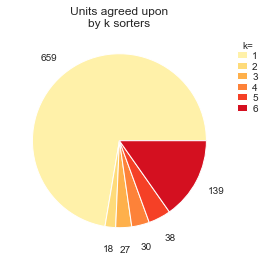In :
```w = sw.plot_multicomp_agreement_by_sorter(mcmp, show_legend=True)
```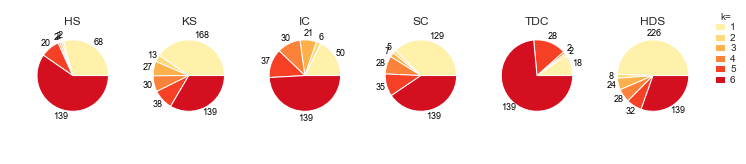In :
```fig = plt.figure(figsize=(14,4))

axes = []
for i,s in enumerate(results['TP'].keys()):
ax = plt.subplot(2,len(sorter_list), i+1)
ax.bar(results['FP'][s].keys(), list(results['FP'][s].values()), alpha=0.5, width = 0.6, color='r', label='false positive')
ax.bar(results['TP'][s].keys(), list(results['TP'][s].values()), bottom=list(results['FP'][s].values()), alpha=0.5, width = 0.6, color='b', label='matched')
ax.set_xticks(range(1,len(sorter_list)+1))
ax.set_xticklabels(range(1,len(sorter_list)+1))
ax.set_title(s)
clear_axes(ax)
axes.append(ax)
if i == 0:
ax.set_ylabel('Number of units')
else:
ax.get_shared_y_axes().join(axes, ax)
ax.set_yticklabels([])

ax = plt.subplot(2,len(sorter_list), len(sorter_list)+i+1)
d  = results['SNR'][s]
sns.boxenplot(data=pd.DataFrame([pd.Series(d[k]) for k in d.keys()]).T, color='b', ax=ax)
ax.set_xticks(range(0,len(sorter_list)))
ax.set_xticklabels(range(1,len(sorter_list)+1))
clear_axes(ax)
axes.append(ax)
if i == 0:
ax.set_ylabel('Ground truth SNR')
ax.set_xlabel('Found by # sorters')
else:
ax.get_shared_y_axes().join(axes, ax)
ax.set_yticklabels([])

```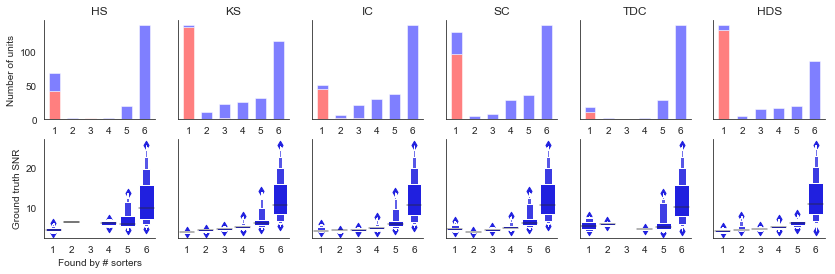In :
```# numbers for figure above

sg_names, sg_units = mcmp.compute_subgraphs()
v, c = np.unique([len(np.unique(s)) for s in sg_names], return_counts=True)
df = pd.DataFrame(np.vstack((v,c,np.round(100*c/np.sum(c),2))).T,
columns=('in # sorters','# units','percentage'))
print('all sorters, all units:')
print(df)
df = pd.DataFrame()
for i, name in enumerate(sorter_names_short):
v, c = np.unique([len(np.unique(sn)) for sn in sg_names if name in sn], return_counts=True)
cl = np.zeros(len(sorter_list), dtype=int)
cl[v.astype(int)-1] = c
df.insert(2*i,name,cl)
df.insert(2*i+1,name+'%',np.round(100*cl/np.sum(cl),1))
print('\nper sorter:')
print(df)

for i,s in enumerate(results['TP'].keys()):
print(s, list(results['FP'][s].values()))
```
```all sorters, all units:
in # sorters  # units  percentage
0           1.0    659.0       72.34
1           2.0     18.0        1.98
2           3.0     27.0        2.96
3           4.0     30.0        3.29
4           5.0     38.0        4.17
5           6.0    139.0       15.26

per sorter:
HS   HS%   KS   KS%   IC   IC%   SC   SC%  TDC  TDC%  HDS  HDS%
0   68  29.2  168  40.5   50  17.7  129  37.6   18   9.5  226  49.5
1    2   0.9   13   3.1    6   2.1    5   1.5    2   1.1    8   1.8
2    2   0.9   27   6.5   21   7.4    7   2.0    0   0.0   24   5.3
3    2   0.9   30   7.2   30  10.6   28   8.2    2   1.1   28   6.1
4   20   8.6   38   9.2   37  13.1   35  10.2   28  14.8   32   7.0
5  139  59.7  139  33.5  139  49.1  139  40.5  139  73.5  139  30.4
HS [42, 1, 2, 0, 1, 0]
KS [164, 1, 2, 0, 1, 0]
IC [45, 2, 2, 0, 0, 0]
SC [97, 1, 0, 0, 1, 0]
TDC [11, 0, 0, 0, 1, 0]
HDS [214, 1, 0, 0, 1, 0]
```

# Ensemble sorting of a Neuropixels recording

## Ensemble sorting of a Neuropixels recording¶

This notebook reproduces figures 1 and 4 from the paper SpikeInterface, a unified framework for spike sorting.

The data set for this notebook is available on the Dandi Archive: https://gui.dandiarchive.org/#/dandiset/000034

The entire data archive can be downloaded with the command `dandi download https://gui.dandiarchive.org/#/dandiset/000034/draft` (about 75GB).

Files required to run the code are:

These files should be in the same directory where the notebook is located (otherwise adjust paths below).

Author: Matthias Hennig, University of Edinburgh, 24 Aug 2020

#### Requirements¶

For this need you will need the following Python packages:

• numpy
• pandas
• matplotlib
• seaborn
• spikeinterface
• dandi
• matplotlib-venn

To run the MATLAB-based sorters, you would also need a MATLAB license. For other sorters, please refer to the documentation on how to install sorters.

In :
```import os

# Matlab sorter paths:
# change these to match your environment
os.environ["IRONCLUST_PATH"] = "./ironclust"
os.environ["KILOSORT2_PATH"] = "./Kilosort2"
os.environ["HDSORT_PATH"] = "./HDsort"

import matplotlib.pyplot as plt
import numpy as np
from pathlib import Path
import pandas as pd
import seaborn as sns
from collections import defaultdict
from matplotlib_venn import venn3

import spikeinterface as si
import spikeextractors as se
import spiketoolkit as st
import spikesorters as ss
import spikecomparison as sc
import spikewidgets as sw
from spikecomparison import GroundTruthStudy, MultiSortingComparison

%matplotlib inline

def clear_axes(ax):
ax.spines['top'].set_visible(False)
ax.spines['right'].set_visible(False)

# print version information
si.print_spikeinterface_version()
ss.print_sorter_versions()
```
```spikeinterface: 0.9.9
* spikeextractor: 0.8.4
* spiketoolkit: 0.6.3
* spikesorters: 0.3.3
* spikecomparison: 0.2.6
* spikewidgets: 0.4.3

hdsort: version = '1.0.2'

herdingspikes: 0.3.7
ironclust: 5.9.8
klusta: 3.0.16
mountainsort4: unknown
spykingcircus: 0.9.7
tridesclous: 1.6.0
```
In :
```# where to find the data set
data_file = Path('./') / 'mouse412804_probeC_15min.nwb'
# results are stored here
study_path = Path('./')
# this folder will contain all results
study_folder = study_path / 'study_15min/'
# this folder will be used as temorary space, and hold the sortings etc.
working_folder = study_path / 'working_15min'

# sorters to use
sorter_list = ['herdingspikes', 'kilosort2', 'ironclust', 'tridesclous', 'spykingcircus', 'hdsort']
# pass the following parameters to the sorters
sorter_params = {
#     'kilosort2': {'keep_good_only': True}, # uncomment this to test the native filter for false positives
'herdingspikes': {'filter': True, }}
sorter_names = ['HerdingSpikes', 'Kilosort2', 'Ironclust','Tridesclous', 'SpykingCircus', 'HDSort']
sorter_names_short = ['HS', 'KS', 'IC', 'TDC', 'SC', 'HDS']
```
In :
```# create an extractor object for the raw data
recording = se.NwbRecordingExtractor(str(data_file))

print("Number of frames: {}\nSampling rate: {}Hz\nNumber of channels: {}".format(
recording.get_num_frames(), recording.get_sampling_frequency(),
recording.get_num_channels()))
```
```Number of frames: 27000000
Sampling rate: 30000.0Hz
Number of channels: 248
```

## Run spike sorters and perform comparison between all outputs¶

In :
```# set up the study environment and run all sorters
# sorters are not re-run if outputs are found in working_folder

if not study_folder.is_dir():
print('Setting up study folder:', study_folder)
os.mkdir(study_folder)

# run all sorters
result_dict = ss.run_sorters(sorter_list=sorter_list, recording_dict_or_list={'rec': recording}, with_output=True,
sorter_params=sorter_params, working_folder=working_folder, engine='loop',
mode='keep', verbose=True)

# store sortings in a list for quick access
sortings = []
for s in sorter_list:
sortings.append(result_dict['rec',s])
```
```/disk/scratch/mhennig/miniconda3/envs/spikesorting/lib/python3.7/site-packages/spikeextractors/extractors/matsortingextractor/matsortingextractor.py:65: ResourceWarning: unclosed file <_io.BufferedReader name='/disk/scratch/mhennig/spikeinterface/Neuropixels_Allen/working_15min/rec/hdsort/hdsort_output/hdsort_output_results.mat'>
raise ImportError("Version 7.2 .mat file given, but you don't have h5py installed.")
ResourceWarning: Enable tracemalloc to get the object allocation traceback
```
In :
```# perform a multi-comparison, all to all sortings
# result is stored, and loaded from disk if the file is found

if not os.path.isfile(study_folder / 'multicomparison.gpickle'):
mcmp = sc.compare_multiple_sorters(sorting_list=sortings, name_list=sorter_names_short,
verbose=True)
print('saving multicomparison')
mcmp.dump(study_folder)
else:
```
```loading multicomparison
Multicomaprison step 3: clean graph
Removed edge ('IC_137', 'HS_49', {'weight': 0.552943287867911})
Removed edge ('KS_185', 'HDS_18005', {'weight': 0.6143539400371452})
Removed edge ('KS_295', 'HS_159', {'weight': 0.6111111111111112})
Removed edge ('KS_195', 'TDC_8', {'weight': 0.6909090909090909})
Removed 4 duplicate nodes
Multicomaprison step 4: extract agreement from graph
```

## Figure 1 - comparison of sorter outputs¶

In :
```# activity levels on the probe

plt.figure(figsize=(16,2))
ax = plt.subplot(111)
w = sw.plot_activity_map(recording, trange=(0,20), transpose=True, ax=ax, background='w', frame=True)
ax.plot((50,150),(-30,-30),'k-')
ax.annotate('100\$\\mu m\$',(100,-90), ha='center');
```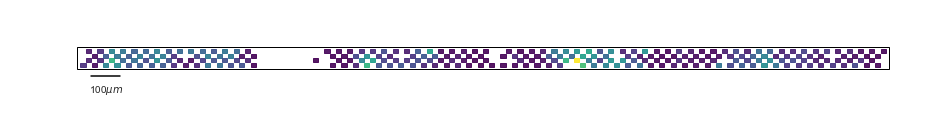In :
```# example data traces

plt.figure(figsize=(16,6))
ax = plt.subplot(111)
w = sw.plot_timeseries(recording, channel_ids=range(20,28), color='k', ax=ax, trange=(1,2))
ax.axis('off')
p = ax.get_position()
p.y0 = 0.55
ax.set_position(p)
ax.set_xticks(())
ax.plot((1.01,1.11),(-1790,-1790),'k-')
ax.annotate('100ms',(1.051,-2900), ha='center');
ax.set_ylim((-2900,ax.set_ylim()))
```
Out:
`(-2900, 19056.0)`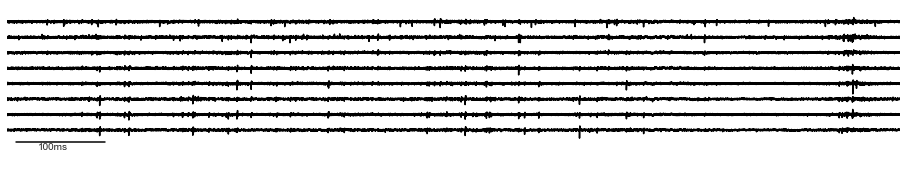In :
```ax = plt.subplot(111)
ax.bar(range(len(sortings)), [len(s.get_unit_ids()) for s in sortings], color='tab:blue')
ax.set_xticks(range(len(sorter_names)))
ax.set_xticklabels(sorter_names_short, rotation=60, ha='center')
ax.set_ylabel('Units detected')
clear_axes(ax)
```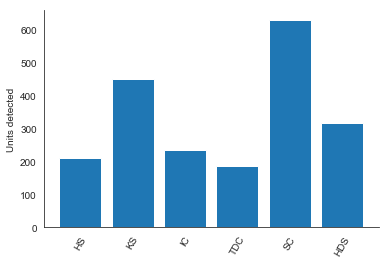In :
```w = sw.plot_multicomp_agreement(mcmp, plot_type='pie')
```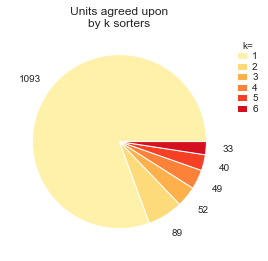In :
```w = sw.plot_multicomp_agreement_by_sorter(mcmp, show_legend=True)
```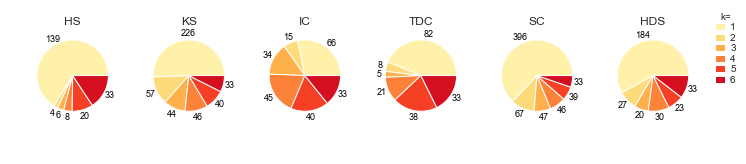In :
```# numbers for figure above

print('number of units detected:')
for i,s in enumerate(sortings):
print("{}: {}".format(sorter_names[i],len(s.get_unit_ids())))

sg_names, sg_units = mcmp.compute_subgraphs()
v, c = np.unique([len(np.unique(s)) for s in sg_names], return_counts=True)
df = pd.DataFrame(np.vstack((v,c,np.round(100*c/np.sum(c),2))).T,
columns=('in # sorters','# units','percentage'))
print('\nall sorters, all units:')
print(df)
df = pd.DataFrame()
for i, name in enumerate(sorter_names_short):
v, c = np.unique([len(np.unique(sn)) for sn in sg_names if name in sn], return_counts=True)
df.insert(2*i,name,c)
df.insert(2*i+1,name+'%',np.round(100*c/np.sum(c),1))
print('\nper sorter:')
print(df)
```
```number of units detected:
HerdingSpikes: 210
Kilosort2: 448
Ironclust: 234
Tridesclous: 187
SpykingCircus: 628
HDSort: 317

all sorters, all units:
in # sorters  # units  percentage
0           1.0   1093.0       80.60
1           2.0     89.0        6.56
2           3.0     52.0        3.83
3           4.0     49.0        3.61
4           5.0     40.0        2.95
5           6.0     33.0        2.43

per sorter:
HS   HS%   KS   KS%  IC   IC%  TDC  TDC%   SC   SC%  HDS  HDS%
0  139  66.2  226  50.7  66  28.3   82  43.9  396  63.1  184  58.0
1    4   1.9   57  12.8  15   6.4    8   4.3   67  10.7   27   8.5
2    6   2.9   44   9.9  34  14.6    5   2.7   47   7.5   20   6.3
3    8   3.8   46  10.3  45  19.3   21  11.2   46   7.3   30   9.5
4   20   9.5   40   9.0  40  17.2   38  20.3   39   6.2   23   7.3
5   33  15.7   33   7.4  33  14.2   33  17.6   33   5.3   33  10.4
```

## Supplemental Figure - example unit templates¶

In :
```# show unit emplates and spike trains for two units/all sorters

sorting = mcmp.get_agreement_sorting(minimum_agreement_count=6)

get_sorting = lambda u: [mcmp.sorting_list[i] for i,n in enumerate(mcmp.name_list) if n==u]
get_spikes = lambda u: [mcmp.sorting_list[i].get_unit_spike_train(u) for i,n in enumerate(mcmp.name_list) if n==u]

# one well matched and one not so well matched unit, all sorters
show_units = [2,17]

for i,unit in enumerate(show_units):
fig = plt.figure(figsize=(16, 2))
ax = plt.subplot(111)
ax.set_title('Average agreement: {:.2f}'.format(sorting.get_unit_property(sorting.get_unit_ids()[unit],'avg_agreement')))
units = sorting.get_unit_property(sorting.get_unit_ids()[unit], 'sorter_unit_ids')
cols = plt.cm.Accent(np.arange(len(units))/len(units))
for j,u in enumerate(dict(sorted(units.items())).items()):
s = get_sorting(u).get_units_spike_train((u,))
s = s[s<20*get_sorting(u).get_sampling_frequency()]
ax.plot(s/get_sorting(u).get_sampling_frequency(), np.ones(len(s))*j, '|', color=cols[j], label=u)
ax.set_frame_on(False)
ax.set_xticks(())
ax.set_yticks(())
ax.plot((0,1),(-1,-1),'k')
ax.annotate('1s',(0.5,-1.75), ha='center')
ax.set_ylim((-2,len(units)+1))

fig = plt.figure(figsize=(16, 2))
units = sorting.get_unit_property(sorting.get_unit_ids()[unit], 'sorter_unit_ids')
print(units)
print('Agreement: {}'.format(sorting.get_unit_property(sorting.get_unit_ids()[unit],'avg_agreement')))
cols = plt.cm.Accent(np.arange(len(units))/len(units))
for j,u in enumerate(dict(sorted(units.items())).items()):
ax = plt.subplot(1, len(sorter_list), j+1)
w = sw.plot_unit_templates(recording, get_sorting(u), unit_ids=(u,), max_spikes_per_unit=10,
lw=1.5, ax=ax, plot_channels=False, set_title=False, axis_equal=True)
# was 100 spikes in original plot
ax.set_title(u)
```
```{'SC': 563, 'TDC': 3, 'HDS': 44005, 'HS': 5, 'KS': 116, 'IC': 88}
Agreement: 0.9661710270732794
{'HS': 49, 'TDC': 15, 'KS': 189, 'HDS': 18005, 'IC': 137, 'SC': 272}
Agreement: 0.6920718057618236
```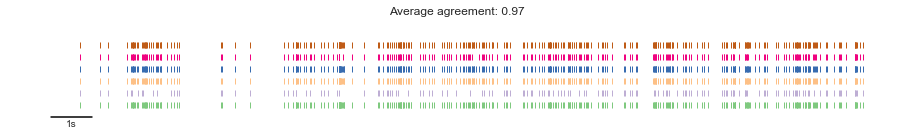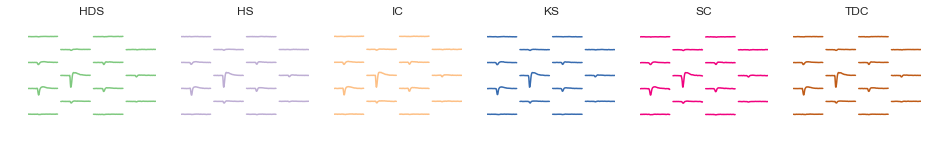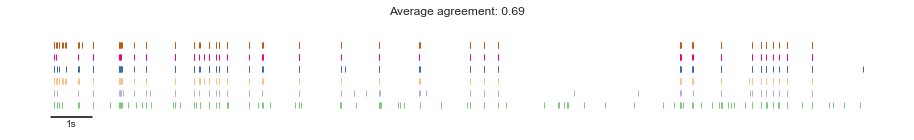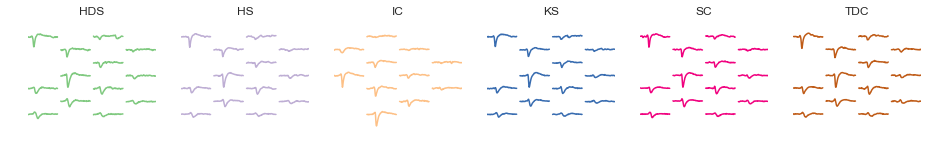## Figure 4 - comparsion between ensembe sortings and curated data¶

In :
```# perform a comparison with curated sortings (KS2)

curated1 = se.NwbSortingExtractor('sub-mouse412804_ses-20200824T155542.nwb', sampling_frequency=30000)
curated2 = se.NwbSortingExtractor('sub-mouse412804_ses-20200824T155543.nwb', sampling_frequency=30000)

comparison_curated = sc.compare_two_sorters(curated1, curated2)
comparison_curated_ks = sc.compare_multiple_sorters((curated1, curated2, sortings[sorter_list.index('kilosort2')]))

# consensus sortings (units where at least 2 sorters agree)
sorting = mcmp.get_agreement_sorting(minimum_agreement_count=2)
consensus_sortings = []
units_dict = defaultdict(list)
units = [sorting.get_unit_property(u,'sorter_unit_ids') for u in sorting.get_unit_ids()]
for au in units:
for u in au.items():
units_dict[u].append(u)
for i,s in enumerate(sorter_names_short):
consensus_sortings.append(se.SubSortingExtractor(sortings[i], unit_ids=units_dict[s]))

# orphan units (units found by only one sorter)
sorting = mcmp.get_agreement_sorting(minimum_agreement_count=1, minimum_agreement_count_only=True)
unmatched_sortings = []
units_dict = defaultdict(list)
units = [sorting.get_unit_property(u,'sorter_unit_ids') for u in sorting.get_unit_ids()]
for au in units:
for u in au.items():
units_dict[u].append(u)
for i,s in enumerate(sorter_names_short):
unmatched_sortings.append(se.SubSortingExtractor(sortings[i], unit_ids=units_dict[s]))

consensus_curated_comparisons = []
for s in consensus_sortings:
consensus_curated_comparisons.append(sc.compare_two_sorters(s, curated1))
consensus_curated_comparisons.append(sc.compare_two_sorters(s, curated2))

unmatched_curated_comparisons = []
for s in unmatched_sortings:
unmatched_curated_comparisons.append(sc.compare_two_sorters(s, curated1))
unmatched_curated_comparisons.append(sc.compare_two_sorters(s, curated2))

all_curated_comparisons = []
for s in sortings:
all_curated_comparisons.append(sc.compare_two_sorters(s, curated1))
all_curated_comparisons.append(sc.compare_two_sorters(s, curated2))    \

# count various types of units

count_mapped = lambda x : np.sum([u!=-1 for u in x.get_mapped_unit_ids()])
count_not_mapped = lambda x : np.sum([u==-1 for u in x.get_mapped_unit_ids()])
count_units = lambda x : len(x.get_unit_ids())

n_consensus_curated_mapped = np.array([count_mapped(c.get_mapped_sorting1()) for c in consensus_curated_comparisons]).reshape((len(sorter_list),2))
n_consensus_curated_unmapped = np.array([count_not_mapped(c.get_mapped_sorting1()) for c in consensus_curated_comparisons]).reshape((len(sorter_list),2))
n_unmatched_curated_mapped = np.array([count_mapped(c.get_mapped_sorting1()) for c in unmatched_curated_comparisons]).reshape((len(sorter_list),2))
n_all_curated_mapped = np.array([count_mapped(c.get_mapped_sorting1()) for c in all_curated_comparisons]).reshape((len(sorter_list),2))
n_all_curated_unmapped = np.array([count_not_mapped(c.get_mapped_sorting1()) for c in all_curated_comparisons]).reshape((len(sorter_list),2))
n_curated_all_unmapped = np.array([count_not_mapped(c.get_mapped_sorting2()) for c in all_curated_comparisons]).reshape((len(sorter_list),2))
n_all = np.array([count_units(s) for s in sortings])
n_consensus = np.array([count_units(s) for s in consensus_sortings])
n_unmatched = np.array([count_units(s) for s in unmatched_sortings])
n_curated1 = len(curated1.get_unit_ids())
n_curated2 = len(curated2.get_unit_ids())
```
In :
```# overlap between two manually curated data and the Kilosort2 sorting they were derived from

i = {}
for k in ['{0:03b}'.format(v) for v in range(1,2**3)]:
i[k] = 0
i['111'] = len(comparison_curated_ks.get_agreement_sorting(minimum_agreement_count=3).get_unit_ids())
s = comparison_curated_ks.get_agreement_sorting(minimum_agreement_count=2, minimum_agreement_count_only=True)
units = [s.get_unit_property(u,'sorter_unit_ids').keys() for u in s.get_unit_ids()]
for u in units:
if 'sorting1' in u and 'sorting2' in u:
i['110'] += 1
if 'sorting1' in u and 'sorting3' in u:
i['101'] += 1
if 'sorting2' in u and 'sorting3' in u:
i['011'] += 1
s = comparison_curated_ks.get_agreement_sorting(minimum_agreement_count=1, minimum_agreement_count_only=True)
units = [s.get_unit_property(u,'sorter_unit_ids').keys() for u in s.get_unit_ids()]
for u in units:
if 'sorting1' in u:
i['100'] += 1
if 'sorting2' in u:
i['010'] += 1
if 'sorting3' in u:
i['001'] += 1
colors = plt.cm.RdYlBu(np.linspace(0,1,3))
venn3(subsets = i,set_labels=('Curated 1', 'Curated 2', 'Kilosort2'),
set_colors=colors, alpha=0.6, normalize_to=100)
```
Out:
`<matplotlib_venn._common.VennDiagram at 0x7fadfef520b8>`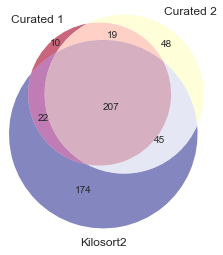In :
```# overlaps betweem ensemble sortings (per sorter) and manually curated sortings

def plot_mcmp_results(data, labels, ax, ylim=None, yticks=None, legend=False):
angles = (np.linspace(0, 2*np.pi, len(sorter_list), endpoint=False)).tolist()
angles += angles[:1]
for i,v in enumerate(data):
v = v.tolist() + v[:1].tolist()
ax.bar(np.array(angles)+i*2*np.pi/len(sorter_list)/len(data)/2-2*np.pi/len(sorter_list)/len(data)/4,
v, label=labels[i],
alpha=0.8, width=np.pi/len(sorter_list)/2)

ax.set_thetagrids(np.degrees(angles), sorter_names_short)
if legend:
ax.legend(bbox_to_anchor=(1.0, 1), loc=2, borderaxespad=0., frameon=False, fontsize=8, markerscale=0.25)
ax.set_theta_offset(np.pi / 2)
ax.set_theta_direction(-1)
if ylim is not None:
ax.set_ylim(ylim)
if yticks is not None:
ax.set_yticks(yticks)

plt.figure(figsize=(14,3))
sns.set_palette(sns.color_palette("Set1"))
ax = plt.subplot(131, projection='polar')
plot_mcmp_results((n_all_curated_mapped[:,0]/n_all*100,
n_all_curated_mapped[:,1]/n_all*100),
('Curated 1','Curated 2'), ax, yticks=np.arange(20,101,20))
ax.set_title('Percent all units\nwith match in curated sets',pad=20);
plt.ylim((0,100))

ax = plt.subplot(132, projection='polar')
plot_mcmp_results((n_consensus_curated_mapped[:,0]/n_consensus*100,
n_consensus_curated_mapped[:,1]/n_consensus*100),
('Curated 1','Curated 2'), ax, yticks=np.arange(20,101,20))
ax.set_title('Percent consensus units\nwith match in curated sets',pad=20);
plt.ylim((0,100))

ax = plt.subplot(133, projection='polar')
plot_mcmp_results((n_unmatched_curated_mapped[:,0]/n_unmatched*100,
n_unmatched_curated_mapped[:,1]/n_unmatched*100),
('Curated 1','Curated 2'), ax, ylim=(0,30), yticks=np.arange(10,21,10), legend=True)
ax.set_title('Percent non-consensus units\nwith match in curated sets',pad=20);
```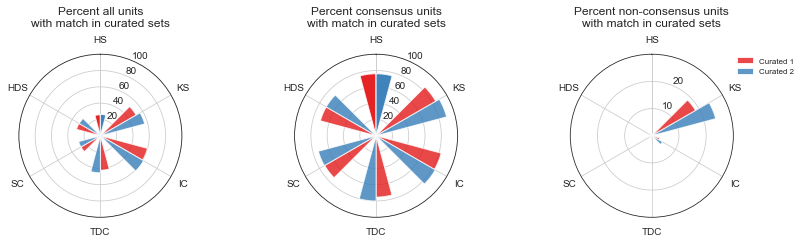In :
```# numbers for figure above

df = pd.DataFrame(np.vstack((n_all_curated_mapped[:,0]/n_all*100, n_all_curated_mapped[:,1]/n_all*100,
n_all_curated_mapped[:,0], n_all_curated_mapped[:,1])).T,
columns = ('C1 %', 'C2 %', 'C1', 'C2'), index=sorter_names_short)
print('Percent all units with match in curated sets')
print(df)

df = pd.DataFrame(np.vstack((n_consensus_curated_mapped[:,0]/n_consensus*100, n_consensus_curated_mapped[:,1]/n_consensus*100,
n_consensus_curated_mapped[:,0],n_consensus_curated_mapped[:,1])).T,
columns = ('C1 %', 'C2 %', 'C1', 'C2'), index=sorter_names_short)
print('\nPercent consensus units with match in curated sets')
print(df)

df = pd.DataFrame(np.vstack((n_unmatched_curated_mapped[:,0]/n_unmatched*100,
n_unmatched_curated_mapped[:,1]/n_unmatched*100,
n_unmatched_curated_mapped[:,0],n_unmatched_curated_mapped[:,1])).T,
columns = ('C1 %', 'C2 %', 'C1', 'C2'), index=sorter_names_short)
print('\nPercent non-consensus units with match in curated sets')
print(df)
```
```Percent all units with match in curated sets
C1 %       C2 %     C1     C2
HS   25.714286  26.190476   54.0   55.0
KS   50.669643  56.250000  227.0  252.0
IC   59.829060  61.111111  140.0  143.0
TDC  42.245989  45.454545   79.0   85.0
SC   27.547771  27.547771  173.0  173.0
HDS  30.283912  29.968454   96.0   95.0

Percent consensus units with match in curated sets
C1 %       C2 %     C1     C2
HS   76.056338  76.056338   54.0   54.0
KS   84.545455  89.545455  186.0  197.0
IC   82.634731  83.832335  138.0  140.0
TDC  75.238095  80.000000   79.0   84.0
SC   72.844828  73.275862  169.0  170.0
HDS  70.676692  70.676692   94.0   94.0

Percent non-consensus units with match in curated sets
C1 %       C2 %    C1    C2
HS    0.000000   0.719424   0.0   1.0
KS   18.584071  24.336283  42.0  55.0
IC    3.030303   4.545455   2.0   3.0
TDC   0.000000   1.219512   0.0   1.0
SC    1.010101   0.757576   4.0   3.0
HDS   1.630435   1.086957   3.0   2.0
```
In [ ]:
```
```

# Marques-Smith neuropixel 384ch paired recording

## Sorter comparison with paired (neuropixel - patch) recordings¶

Author : Samuel Garcia

André Marques-Smith make an open dataset with simultaneous patch-clamp and neuropixel probe extracellular recordings from the same cortical neuron in anaesthetized rats.

This is very very usefull to test spike sorting engine.

The original contain 42 recordings.

Here we select only a subset of 6 files. I keep only when the SNR in the extra cellular trace is big enough to be detected. One file (c24) was remove because the juxta cellular itself is ambiguous.

The patch recording will be the "Groud Truth". And the neuropixel with 384ch will computed by 5 sorters to compare results.

Please have a look to the paper:

https://www.biorxiv.org/content/10.1101/370080v2

The repo the explain everything

https://github.com/kampff-lab/sc.io/tree/master/Paired%20Recordings

Data set availaible here :

http://crcns.org/data-sets/methods/spe-1

or here

Note :

• I will note use the spiek indexes provide by André because for some files small errors on double peak detection can occurs.
• This results is also on spike forest here but the spikeforest keep only 32 channels to reduce the computation. Here the computation is done on 384 channels. Lets see if we have the same results.
In :
```# import everything
import os, getpass

kilosort2_path = '/home/samuel/Documents/Spikeinterface/Kilosort2'
os.environ["KILOSORT2_PATH"] = kilosort2_path

kilosort_path = '/home/samuel/Documents/Spikeinterface/KiloSort/'
os.environ["KILOSORT_PATH"] = kilosort_path

ironclust_path = '/home/samuel/Documents/Spikeinterface/ironclust'
os.environ["IRONCLUST_PATH"] = ironclust_path

from pathlib import Path
import scipy.signal
import scipy.io

import numpy as np
import pandas as pd
import matplotlib.pyplot as plt
import seaborn as sns
from pathlib import Path

import spikeinterface as si
import spikeinterface.extractors as se
import spikeinterface.sorters as ss
import spikeinterface.widgets as sw

from spikeinterface.comparison import GroundTruthStudy
```
In :
```si.print_spikeinterface_version()
ss.print_sorter_versions()
```
```spikeinterface: 0.9.9
* spikeextractor: 0.8.4
* spiketoolkit: 0.6.3
* spikesorters: 0.3.3
* spikecomparison: 0.2.6
* spikewidgets: 0.4.3

herdingspikes: 0.3.7+git.45665a2b6438
ironclust: 5.9.8
kilosort: git-cd040da1963d
kilosort2: git-e243c934339e
spykingcircus: 0.9.7
tridesclous: 1.6.1.dev
```

### path and list¶

In :
```p = '/media/samuel/dataspikesorting/DataSpikeSortingHD2/andre_paired_neuropixel/'
p = Path(p)

recordings_folder = p / 'recordings'
study_folder = p / 'study_paired_neuropixel'

rec_names = [
'c14',
'c26',
'c28',
'c37',
'c45',
'c46'
]
```

### function to detect peak from patch recording¶

File provide by André contains small errors in peak detection. Here we computed then again.

In :
```def detect_peak_on_patch_sig(patch_sig, sample_rate):
# filter because some traces have drift
sos = scipy.signal.iirfilter(5, 200./sample_rate*2, analog=False, btype = 'highpass', ftype = 'butter', output = 'sos')
patch_sig_f = scipy.signal.sosfiltfilt(sos, patch_sig, axis=0)

med = np.median(patch_sig_f)
thresh = med - 12 * mad

# 1 ms aounrd peak
d = int(sample_rate * 0.001)
spike_indexes, prop = scipy.signal.find_peaks(-patch_sig_f, height=-thresh, distance=d)

#~ fig, ax = plt.subplots()
#~ ax.plot(patch_sig_f)
#~ ax.axhline(thresh)
#~ ax.plot(spike_indexes, patch_sig_f[spike_indexes], ls='None', marker='o')
#~ plt.show()

return spike_indexes
```
In [ ]:
```## create the study
```
In [ ]:
```# the file chanMap.mat contain the geometry
locations = np.zeros((384, 2))
locations[:, 0] = d['xcoords'][:, 0]
locations[:, 1] = d['ycoords'][:, 0]

#~ fig, ax = plt.subplots()
#~ ax.scatter(locations[:, 0], locations[:, 1])
#~ plt.show()

gt_dict = {}
for rec_name in rec_names:
print(rec_name)
sample_rate = 30000.

# neuropixel sigs
raw_bin_filename = recordings_folder /  rec_name / (rec_name + '_npx_raw.bin')
mea_sigs = np.memmap(raw_bin_filename, dtype='int16', mode='r').reshape(-1, 384)

# patch recoring
filename = recordings_folder /  rec_name / (rec_name + '_patch_ch1.bin')
#~ patch_sig = np.memmap(str(filename), dtype='float64', mode='r')
patch_sig = np.fromfile(str(filename), dtype='float64')

# spike index inn the patch clock refrence
sr = 50023. #  this is not theexact freq but it do not matter here
gt_spike_indexes_patch = detect_peak_on_patch_sig(patch_sig, sr)

# this is time factor strech between the 2 recordings (neuropixel and patch)
time_factor = mea_sigs.shape / patch_sig.shape
print('time_factor', time_factor)

# spike index in the neuropixel clock refrence
gt_spike_indexes = (gt_spike_indexes_patch * time_factor).astype('int64')

# recording
rec = se.BinDatRecordingExtractor(raw_bin_filename, sample_rate, 384, 'int16', offset=0, time_axis=0)
rec.set_channel_locations(locations)

# gt sorting
sorting_gt = se.NumpySortingExtractor()
sorting_gt.set_times_labels(gt_spike_indexes, np.zeros(gt_spike_indexes.size, dtype='int64'))
sorting_gt.set_sampling_frequency(sample_rate)

gt_dict[rec_name] = (rec, sorting_gt)

study = GroundTruthStudy.create(study_folder, gt_dict)
```

### Get signal to noise ratio for all units¶

In [ ]:
```study = GroundTruthStudy(study_folder)
snr = study.concat_all_snr()
snr
```
In [ ]:
```fig, ax = plt.subplots()
ax.hist(snr['snr'].values, bins=np.arange(0, 40, 5))
ax.set_xlabel('GT units SNR')
```

### Run all sorters¶

In :
```sorter_list = ['herdingspikes', 'ironclust', 'kilosort2',
'spykingcircus', 'tridesclous']
```
In [ ]:
```study = GroundTruthStudy(study_folder)

study.run_sorters(sorter_list, mode='keep', verbose=False)
```

### Run comparison with ground truth and retreive result tables¶

In [ ]:
```study = GroundTruthStudy(study_folder)
```
In [ ]:
```# this copy sorting is necessary to copy results from sorter
# into a centralize folder with all results
study.copy_sortings()
```
In [ ]:
```# this run all comparison to GT
# exhaustive_gt=False because it is a pair recording so only one GT units
study.run_comparisons(exhaustive_gt=False, match_score=0.1, overmerged_score=0.2)
```
In [ ]:
```# this retrieve results
comparisons = study.comparisons
dataframes = study.aggregate_dataframes()
```

### Run times¶

In [ ]:
```dataframes['run_times'].set_index(['rec_name', 'sorter_name']).unstack('sorter_name')
```
In [ ]:
```sns.set_palette(sns.color_palette("Set1"))

#fig, ax = plt.subplots()
g = sns.catplot(data=dataframes['run_times'], x='sorter_name', y='run_time',
hue="rec_name",
order=sorter_list,
kind='bar',
legend=False)
g.fig.set_size_inches(12,5)

#ax.set_yscale('log')
#ax.set_ylabel('Run time (s)');
#ax.set_xlabel(None);
fig.axes.set_xticklabels(sorter_list, rotation=40, ha='right');
```

### accuracy/precision/recall scores per sorters¶

In [ ]:
```sns.set_palette(sns.color_palette("Set1"))

df = pd.melt(dataframes['perf_by_units'], id_vars=['rec_name', 'sorter_name'],
var_name='metric', value_name='score',
value_vars=('accuracy','precision', 'recall'))
display(df)

# df.assign(Generation=df.metric.map({'metric':'Metric','accuracy': 'Accuracy', 'precision': 'Precision', 'recall': 'Recall'}))
g = sns.catplot(data=df, x='sorter_name', y='score', hue='metric', kind='swarm', dodge=True, # ax=ax,
order=sorter_list, legend_out=True, s=4)
g.fig.set_size_inches(12,5)
```
In [ ]:
```sns.set_palette(sns.color_palette("deep"))
g = sns.relplot(data=dataframes['perf_by_units'], x='precision', y='recall', col='sorter_name',
col_wrap=3, col_order=sorter_list, s=60)
```

### Accuracy vns SNR¶

In [ ]:
```df = dataframes['perf_by_units']
df
```
In [ ]:
```df = dataframes['perf_by_units']

# add snr to the by-unit table
df['snr'] = None
for rec_name, gt_id in snr.index:
df['snr'].loc[(df['gt_unit_id']==gt_id) & (df['rec_name']==rec_name)] = snr.at[(rec_name, gt_id), 'snr']

sns.set_palette(sns.color_palette("deep"))
g = sns.relplot(data=df, x='snr', y='accuracy', col='sorter_name',
col_wrap=3, col_order=sorter_list, s=80)
```

# Does the recording's duration affect the quality of spike sorting?

## Does the recording's duration affect the quality of spike sorting?¶

This notebook investigates if and how the duration of the recording affects spike sorting.

Obviously, each sorter engine needs a minimum number of events to detect a "cluster", and therefore a unit. If a neuron doesn't fire enough during a recording it won't be detected. The number of event per units depends on the recording duration and the each individual firing rates.

In order to test this phenomenon, we use the same dataset (with the same neurons and firing rates), but we vary the duration of the recording.

The simulated recording is generated with MEArec using a Neuronexus-32 probe. This specific dataset seems relatively easy to sort. The "SYNTH_MEAREC_NEURONEXUS" dataset in SpikeForest (which uses the same probe), in fact, shows quite good results for all sorters. The original duration is 600s (10 min).

Here we have generated a new but similar recording with a duration of 1800s. Then we have shortened it to 60s, 300s, 600s and 1800s (original). The recording can be downloaded from Zenodo: https://doi.org/10.5281/zenodo.4058272

The dataset name is: recordings_10cells_Neuronexus-32_1800.0_10.0uV_2020-02-28.h5. It contains 10 neurons recorded on a Neuronexus-32 probe. The duration is 1800s and the noise level is 10uV.

Let's see if spike sorters are robust to fewer events and if are able to deal with long durations or they end up finding too many events.

Author: Samuel Garcia, CRNL, Lyon

#### Requirements¶

For this need you will need the following Python packages:

• numpy
• pandas
• matplotlib
• seaborn
• spikeinterface

To run the MATLAB-based sorters, you would also need a MATLAB license. For other sorters, please refer to the documentation on how to install sorters.

#### Installation and imports¶

In :
```import os
import numpy as np
import pandas as pd
import matplotlib.pyplot as plt
import seaborn as sns
from pathlib import Path

import spikeinterface as si
import spikeinterface.extractors as se
import spikeinterface.sorters as ss
import spikeinterface.widgets as sw

from spikeinterface.comparison import GroundTruthStudy
```
```/home/samuel/.virtualenvs/py36/lib/python3.6/site-packages/statsmodels/tools/_testing.py:19: FutureWarning: pandas.util.testing is deprecated. Use the functions in the public API at pandas.testing instead.
import pandas.util.testing as tm
```
In [ ]:
```# clone and install MATLAB sorters

# kilosort2
!git clone https://github.com/MouseLand/Kilosort2.git
kilosort2_path = './Kilosort2'
ss.Kilosort2Sorter.set_kilosort2_path(kilosort2_path)

# kilosort
!git clone https://github.com/cortex-lab/KiloSort.git
kilosort_path = './KiloSort'
ss.KilosortSorter.set_kilosort_path(kilosort_path)

# ironclust
!git clone https://github.com/flatironinstitute/ironclust.git
ironclust_path = './ironclust'
ss.IronclustSorter.set_ironclust_path(ironclust_path)
```
In :
```%matplotlib inline

# some matplotlib hack to prettify figure
SMALL_SIZE = 12
MEDIUM_SIZE = 14
BIGGER_SIZE = 16

plt.rc('font', size=SMALL_SIZE)          # controls default text sizes
plt.rc('axes', titlesize=SMALL_SIZE)     # fontsize of the axes title
plt.rc('axes', labelsize=MEDIUM_SIZE)    # fontsize of the x and y labels
plt.rc('xtick', labelsize=SMALL_SIZE)    # fontsize of the tick labels
plt.rc('ytick', labelsize=SMALL_SIZE)    # fontsize of the tick labels
plt.rc('legend', fontsize=SMALL_SIZE)    # legend fontsize
plt.rc('figure', figsize=(10.0, 8.0))  # figsize
plt.rc('figure', titlesize=BIGGER_SIZE)  # fontsize of the figure title

def clear_axes(ax):
ax.spines['top'].set_visible(False)
ax.spines['right'].set_visible(False)
```

### Check spikeinterface version and sorter version¶

In :
```si.print_spikeinterface_version()
ss.print_sorter_versions()
```
```spikeinterface: 0.9.1
* spikeextractor: 0.7.2
* spiketoolkit: 0.5.2
* spikesorters: 0.2.4
* spikecomparison: 0.2.3
* spikewidgets: 0.3.3

herdingspikes: 0.3.7+git.45665a2b6438
ironclust: 5.9.4
kilosort: git-cd040da1963d
kilosort2: git-67a42a87b866
klusta: 3.0.16
mountainsort4: unknown
spykingcircus: 0.9.2
tridesclous: 1.5.0
```

### Setup global path¶

In :
```# Change this path to point to where you downloaded the dataset
p = Path('/home/samuel/Documents/DataSpikeSorting/mearec/')
study_folder = p / 'study_mearec_neuronexus_several_durations/'
```

### Setup ground truth study¶

In :
```mearec_filename = p / 'recordings_10cells_Neuronexus-32_1800.0_10.0uV_2020-02-28.h5'
```
In [ ]:
```rec  = se.MEArecRecordingExtractor(mearec_filename, locs_2d=True)
gt_sorting = se.MEArecSortingExtractor(mearec_filename)

fs = rec.get_sampling_frequency()

gt_dict = {}
durations = [60, 300, 600, 1800]
for duration in durations:
sub_rec = se.SubRecordingExtractor(rec, start_frame=0, end_frame=int(duration*fs))
sub_sorting = se.SubSortingExtractor(gt_sorting, start_frame=0, end_frame=int(duration*fs))
gt_dict[f'rec{duration}'] = (sub_rec, sub_sorting)
study = GroundTruthStudy.create(study_folder, gt_dict)
```

### Run all sorters¶

In :
```sorter_list = ['herdingspikes', 'ironclust', 'kilosort2',  'kilosort',
'mountainsort4', 'spykingcircus', 'tridesclous']
```
In [ ]:
```study = GroundTruthStudy(study_folder)
sorter_params = {}
study.run_sorters(sorter_list, sorter_params=sorter_params, mode='keep', verbose=True)
```

### Get signal to noise ratio for all units¶

Units are the same in each recording so the snr is the same lets take from the longest one

In :
```study = GroundTruthStudy(study_folder)
snr = study.get_units_snr```